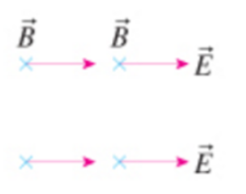# Problem: In what direction is the electromagnetic wave traveling in the figure (Figure 2) b? a. To the right b. into the page c. Out of the page d. Downward e. Upward f. To the left

###### FREE Expert Solution

The electromagnetic wave always moves in a direction perpendicular to both electric and magnetic fields.

is the electric field vector and B is the magnetic field vector.

The vector product of E and B gives the direction of electromagnetic wave motion.

83% (237 ratings)###### Problem Details

In what direction is the electromagnetic wave traveling in the figure (Figure 2) b?

a. To the right

b. into the page

c. Out of the page

d. Downward

e. Upward

f. To the left